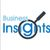# QlikView Documents

QlikView documentation and resources.

Announcements
Our next Qlik Insider session will cover new key capabilities. Join us August 11th REGISTER TODAY

# How to use - Dimensionality()MVP

## How to use - Dimensionality()

Definition:

Returns the number of dimension columns that have non-aggregation content. i.e. do not contain partial sums or collapsed aggregates.

A typical use is in attribute expressions, when you want to apply different cell formatting depending on aggregation level of data.

This function is only available in charts. For all chart types except pivot table it will return the number of dimensions in all rows except the total, which will be 0.

What does it mean?

We have Table with 4 dimensions(columns): Product,Category,Type,Sales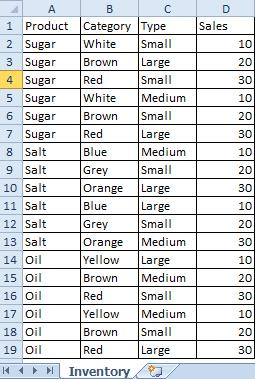Now we want to create Pivot Table by using those Dimensions.

We are going to use only 3 of them(Product,Category,Type) and use 4th(Sales) in our expression.

The result is shown below: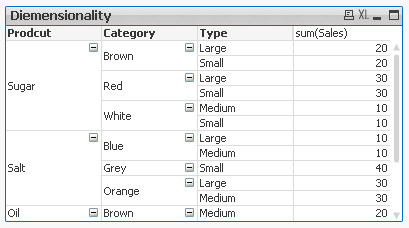This Pivot Table  has 3 dimensions so its maximum dimensionality is 3.

For better understating please see  table below.

The function is used to show on which dimensionality level each of the Pivot Table row is: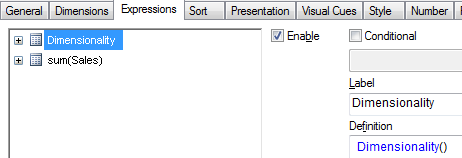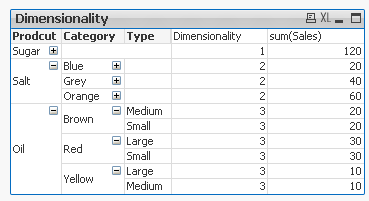'Sugar' has dimensionality of 1 which is Total for that 'Product'.

'Salt' has dimensionality of 2 which is Total for each 'Category' of that 'Product'.

'Oil' has dimensionality of 3 which is single value for each 'Type' of the 'Product's' 'Category'.

So then more Dimension we use the greater dimensionality of our Pivot Table is.

Practical use:

1) To show the level of dimensionality: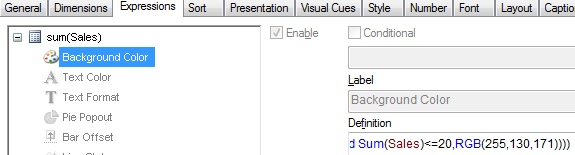Expression:

if(Dimensionality()=1 ,RGB(151,255,255),if(Dimensionality()=2 ,RGB(0,238,0),if(Dimensionality()=3,RGB(255,130,171))))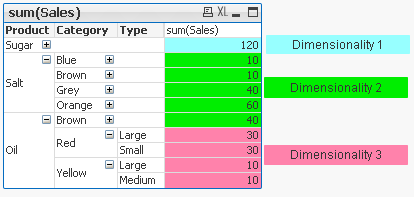2) Highlight background of rows which on each level  fall into certain condition:

Expression:

if(Dimensionality()=1 and sum(Sales)<150,RGB(151,255,255),if(Dimensionality()=2 and sum(Sales)<=20,RGB(0,238,0),if(Dimensionality()=3 and Sum(Sales)<=20,RGB(255,130,171))))

LEVEL1 --> Values <140LEVEL 2 --> Values <=20LEVEL 3 --> Values <=20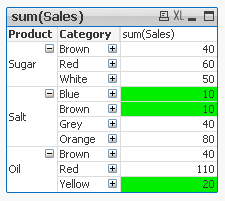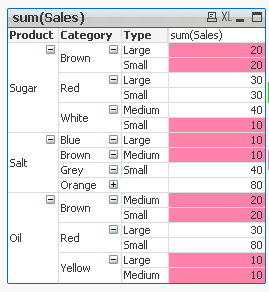• To use the script below the Excel file must be saved in the same folder that your qvd file

Otherwise you will need to make changes the this path - [Dimensionality.xlsx]

Directory;

Category,

Type,

Sales

FROM

[Dimensionality.xlsx]

(ooxml, embedded labels, table is Sheet1);

Felling Qlingry?

How To /Missing Manual (25 articles)

Labels (3)

• ### Layout & Visualizations